First Law Of Thermodynamic MCQ Level - 1 (Part - 1)

# First Law Of Thermodynamic MCQ Level - 1 (Part - 1)

Test Description

## 10 Questions MCQ Test Topic wise Tests for IIT JAM Physics | First Law Of Thermodynamic MCQ Level - 1 (Part - 1)

First Law Of Thermodynamic MCQ Level - 1 (Part - 1) for IIT JAM 2023 is part of Topic wise Tests for IIT JAM Physics preparation. The First Law Of Thermodynamic MCQ Level - 1 (Part - 1) questions and answers have been prepared according to the IIT JAM exam syllabus.The First Law Of Thermodynamic MCQ Level - 1 (Part - 1) MCQs are made for IIT JAM 2023 Exam. Find important definitions, questions, notes, meanings, examples, exercises, MCQs and online tests for First Law Of Thermodynamic MCQ Level - 1 (Part - 1) below.
Solutions of First Law Of Thermodynamic MCQ Level - 1 (Part - 1) questions in English are available as part of our Topic wise Tests for IIT JAM Physics for IIT JAM & First Law Of Thermodynamic MCQ Level - 1 (Part - 1) solutions in Hindi for Topic wise Tests for IIT JAM Physics course. Download more important topics, notes, lectures and mock test series for IIT JAM Exam by signing up for free. Attempt First Law Of Thermodynamic MCQ Level - 1 (Part - 1) | 10 questions in 30 minutes | Mock test for IIT JAM preparation | Free important questions MCQ to study Topic wise Tests for IIT JAM Physics for IIT JAM Exam | Download free PDF with solutions
 1 Crore+ students have signed up on EduRev. Have you?
First Law Of Thermodynamic MCQ Level - 1 (Part - 1) - Question 1

### A vessel contains 1 mole of O2 gas (molar mass 32) at a temperature T. The pressure of the gas is p. An identical vessels containing one mole of the gas (molar mass 4) at a temperature 2T has a pressure of

Detailed Solution for First Law Of Thermodynamic MCQ Level - 1 (Part - 1) - Question 1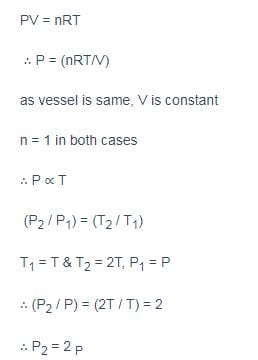First Law Of Thermodynamic MCQ Level - 1 (Part - 1) - Question 2

### A gas mixture consists of 2 moles of oxygen and 4 moles of argon at temperature T. Neglecting all vibrational modes, the total internal energy of the system is

Detailed Solution for First Law Of Thermodynamic MCQ Level - 1 (Part - 1) - Question 2

Internal energy of n moles of an ideal gas at temperature T is given by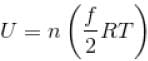where, f = degrees of freedom
= 5 for O3 and 3 for Ar  [∴ O2 is diatomic, Ar is monoatomic]
Hence,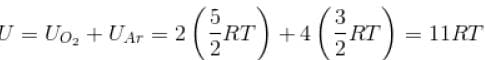The correct answer is: 11 RT

First Law Of Thermodynamic MCQ Level - 1 (Part - 1) - Question 3

### An ideal monoatomic gas is taken round that cycle ABCDA as shown in the p-V diagram (see figure). The work done during the cycle is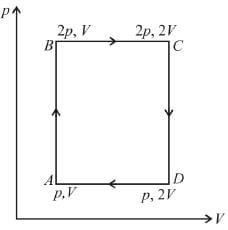Detailed Solution for First Law Of Thermodynamic MCQ Level - 1 (Part - 1) - Question 3

Work done in a cyclic process = area under the graph in p-V diagram
Area = AB × BC = (2p – p) × (2V – V) = pV
∴ So, work done = pV

First Law Of Thermodynamic MCQ Level - 1 (Part - 1) - Question 4

In a given process of an ideal gas, dW = 0 and dQ < 0. The for the gas
Select one:

Detailed Solution for First Law Of Thermodynamic MCQ Level - 1 (Part - 1) - Question 4

From first law of thermodynamics,
dQ = dU + dW
dQ = dU if dW = 0
Since, dQ < 0
Therefore,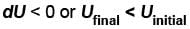or temperature will decrease
The correct answer is: the temperature will decrease

First Law Of Thermodynamic MCQ Level - 1 (Part - 1) - Question 5

70 cal of heat are required to raise the temperature of 2 moles of an ideal diatomic gas at constant pressure from 30ºC to 35°C. The amount of heat required (in calorie) to raise the temperature of the same gas through the same range (30°C to 35°C) at constant volume is

Detailed Solution for First Law Of Thermodynamic MCQ Level - 1 (Part - 1) - Question 5

We know that heat required to raise the temperature at constant pressure is Cp.
So,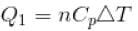Similarly, at constant volume CV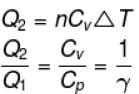or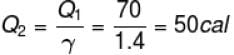First Law Of Thermodynamic MCQ Level - 1 (Part - 1) - Question 6

An ideal gas is initially at temperature T and volume V. Its volume is increased by ΔV  due to an increase in temperature ΔT  pressure remaining constant. The quantity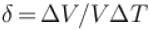varies with temperature as
Select one:

Detailed Solution for First Law Of Thermodynamic MCQ Level - 1 (Part - 1) - Question 6

For an ideal gas : pV = nRT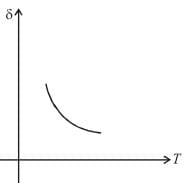For p = constant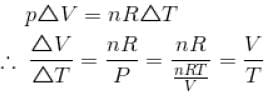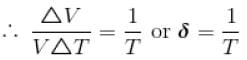Therefore,δ is inversely proportional to temperature T i.e., when T increases, δ decreases and vice-versa.
Hence,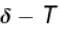graph will be rectangular hyperbola as shown in the above figure.
The correct answer is: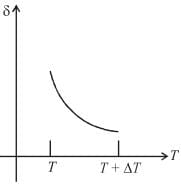First Law Of Thermodynamic MCQ Level - 1 (Part - 1) - Question 7

When an ideal diatomic gas is heated at constant pressure, the fraction of the heat energy supplied  which increases the internal energy of the gas is
Select one:

Detailed Solution for First Law Of Thermodynamic MCQ Level - 1 (Part - 1) - Question 7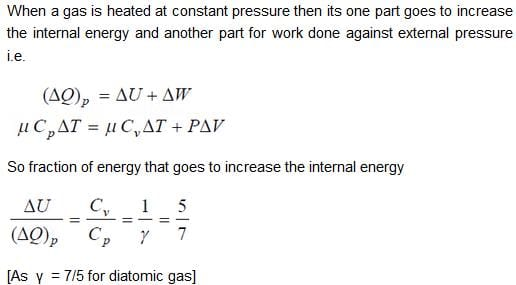First Law Of Thermodynamic MCQ Level - 1 (Part - 1) - Question 8

Starting with the same initial conditions, an ideal gas expands from volume V1 to V2 in three different ways, the work done by the gas is W1 if the process is purely isothermal, W2 if purely isobaric and W3 if purely adiabatic, then

Detailed Solution for First Law Of Thermodynamic MCQ Level - 1 (Part - 1) - Question 8

The corresponding p-V graph (also called indicator diagram) in three different process will be as shown :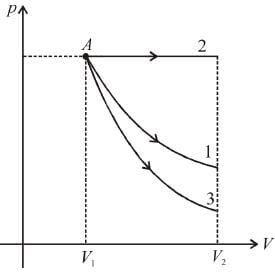Area under the graph gives the work done by the gas.
(Area)2 > (Area)1 > (Area)3
∴ W2 > W1 > W3
W2 > W1 > W3

First Law Of Thermodynamic MCQ Level - 1 (Part - 1) - Question 9

An ideal gas expands isothermally from a volume V1 to V2 and then compressed to original volumes V1 adiabatically. Initial pressure is p1 and final pressure is p3. The total work done is W. Then,
Select one:

Detailed Solution for First Law Of Thermodynamic MCQ Level - 1 (Part - 1) - Question 9

Slope of adiabatic process at a given state (p, V, T) is more than the slope of isothermal process. The corresponding p-V graph for the two process is as shown in figure.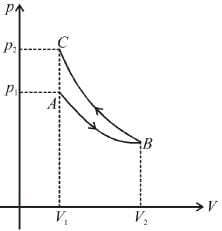In the graph, AB is isothermal and BC is adiabatic.
WAB is positive (as volume is increasing)
and WBC is negative (as volume is decreasing)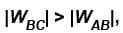as area under p–V graph gives the work done.
Hence,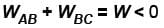From the graph itself, it is clear that p3 > p1
The correct answer is: p> p1 , W < 0

First Law Of Thermodynamic MCQ Level - 1 (Part - 1) - Question 10

Steam at 100°C is passed into 1.1 kg of water contained in a calorimeter of water equivalent 0.02 kg at 15°C till the temperature of the calorimeter and its contents rises to 80°C. The mass of the steam condensed (in kg) is
Select one:

Detailed Solution for First Law Of Thermodynamic MCQ Level - 1 (Part - 1) - Question 10

Heat lost by steam =Heat gained by
(water +calorimeter)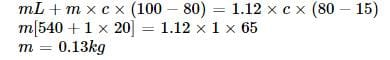## Topic wise Tests for IIT JAM Physics

217 tests
 Use Code STAYHOME200 and get INR 200 additional OFF Use Coupon Code
Information about First Law Of Thermodynamic MCQ Level - 1 (Part - 1) Page
In this test you can find the Exam questions for First Law Of Thermodynamic MCQ Level - 1 (Part - 1) solved & explained in the simplest way possible. Besides giving Questions and answers for First Law Of Thermodynamic MCQ Level - 1 (Part - 1), EduRev gives you an ample number of Online tests for practice

217 tests